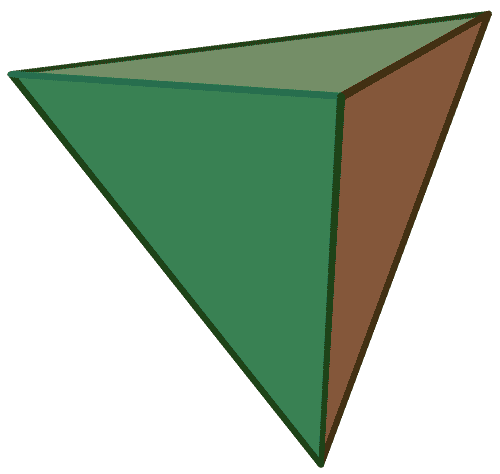# Geometry and Trigonometry Symbols

A comprehensive collection of the most common symbols in geometry and trigonometry, categorized by function into tables along with each symbol's term, meaning and example.

Geometry and trigonometry are branches of mathematics concerned with geometrical figures and angles of triangles. The following list documents some of the most notable symbols in these topics, along with each symbol’s usage and meaning.

For readability purpose, these symbols are categorized by their function into tables. Other comprehensive lists of math symbols — as categorized by subject and type — can be also found in the relevant pages below (or in the navigational panel).

Get the master summary of mathematical symbols in eBook form — along with each symbol’s usage and LaTeX code.

## Point/Line-related Symbols

In geometry, points and lines form the foundation of more complex geometrical figures such as triangles, circles, quadrilaterals and polygons. The following table documents some of the most notable symbols related to these — along with each symbol’s meaning and example.

## Angle-related Symbols

An angle essentially corresponds to an “opening” of a geometrical figure, whose quantification leads to much development in geometry and trigonometry. The following table documents some of the most notable symbols related to angles — along with each symbol’s meaning and example.

## Circle-related Symbols

A circle can be thought of as a set of all points equidistant to a given point, and often plays a crucial role in the development of Euclidean geometry and trigonometry. The following table documents some of the most notable symbols related to circle — along their respective meaning and example.

## Trigonometric Functions

In trigonometry, many functions are used to relate angles within a right triangle to its various lengths or ratios. The following table documents some of the most common functions in this category — along with their respective usage and example.

## Other 2D/3D-figure-related Symbols

In elementary geometry, much of the study revolves around the analysis of polygons, polyhedra and other 3-dimensional figures. The following table documents some of the most notable symbols in these categories — along with each symbol’s respective meaning and usage.

The following figures illustrate the 5 platonic solids (regular, convex polyhedra), along with their respective number of vertices, edges and faces.Tetrahedron $(V= 4, E= 6, \\ F=4, \chi=2)$

For the master list of symbols, see mathematical symbols. For lists of symbols categorized by type and subject, refer to the relevant pages below for more.

1.